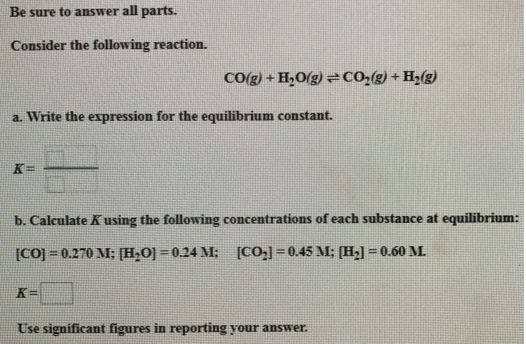# Problem: Consider the following reaction. CO(g) + H2O(g) ⇌ CO2(g) + H2(g) a. Write the expression for the equilibrium constant. b. Calculate K using the following concentrations of each substance at equilibrium: [CO] = 0.270 M; [H2O] = 0.24 m; [CO2] = 0.45 M; [H2] = 0.60 M.

###### FREE Expert Solution
99% (276 ratings)###### Problem Details

Consider the following reaction.

CO(g) + H2O(g) ⇌ CO2(g) + H2(g)

a. Write the expression for the equilibrium constant.

b. Calculate K using the following concentrations of each substance at equilibrium:

[CO] = 0.270 M; [H2O] = 0.24 m; [CO2] = 0.45 M; [H2] = 0.60 M.